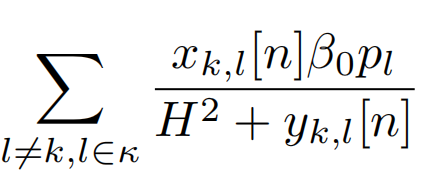# Problem of disciplined convex programming error: Illegal operation: log( {convex} )

Hi everyone,
I got an error message when I use cvx in my code. So, I need your help. The problem is as follows:HOW TO WRITE THIS IN DCP ?

where p, H^2, \beta0 is constant, x_{k,l}[n], y_{k,l}[n] both are a three-dimensional array. But x_{k,l}[n] in this block is a definite value, so we also can regard it as a constant.

I would be grateful if you could answer my questions. Thanks, everyone.

If you got an error message Illegal operation: log( {convex} ) , then you obviously are not showing all of your pertinent code. My mention of inv_pos is based on there not being any log… If there is log somewhere, you need to show it. Expressions of the form x*log(1+y/x) and other variations can be reformulated to be accepted by CVX.

help inv_pos

inv_pos Reciprocal of a positive quantity.
inv_pos(X) returns 1./X if X is positive, and +Inf otherwise.
X must be real.

 For matrices and N-D arrays, the function is applied to each element.

Disciplined convex programming information:
inv_pos is convex and nonincreasing; therefore, when used in CVX
specifications, its argument must be concave (or affine).

Thank you !！
This is the complete code.

My initial idea was to use the for loop to achieve this sum, but it was not possible. So I want to ask how to use the DCP specification to implement this code.

This is the code of using for loop.

Use log_sum_exp in the first approach in section 5.2.7 Log-sum-inv of 5 Exponential cone optimization — MOSEK Modeling Cookbook 3.3.0 .

And in the future, please copy and paste code using Preformattet text icon, rather than posting an image.